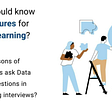# Mathematics and Algorithms

Albert Einstein who is a mathematician at first before being a physicist. said, “Pure mathematics is, in its way, the poetry of logical ideas”.

In the past, education of mathematic and physic was happing in the same departments. Scientists were talking about pure and applied mathematics for centuries. Then, different fields of science were born from mathematics. But we are still talking about pure and applied mathematics. In order not to burn the ashes of controversy again, I immediately give up physics examples. Anyway, what is the difference between pure and applied mathematics? For example, Number theory or arithmetic or higher arithmetic in older usage is a branch of pure mathematics devoted primarily to the study of the integers. But Number Theory is mostly applied, for example, in modern Data Encryption Techniques (cryptography).

Dean Schlicter said “Go down deep enough into anything and you will find mathematics.”

When we look more generally, the computers are an output of applied mathematics. Operating on computers requires a systematic process. We call the planning part of this systematic process an algorithm. We typically want to solve a class of problems or to perform a computation. The definition of an algorithm is the same in mathematics and computer science too. Besides, advances in pure mathematics continue to contribute to computer science every day, just like other applied sciences.

Galileo Galilei said “Mathematics is the language with which God has written the universe.”

Every algorithm has two dimensions to evaluate. One is how complicated, second is how fast it is. Besides, the complexity depends on the quality of the solution. For example, now, we will examine the multiplication algorithm. As you know, we use the decimal system in daily life. This comes from using different ten figures or numbers from 0 to 9. But, the computer uses a binary system. So there are only 2 numbers, 0 and 1.

You might ask why binary system? The answer is simple: Hardware and laws of physics. Every number in your computer is an electrical signal, and in the early days of computers, it was much more difficult to measure and control electrical signals with great precision. It made more sense to distinguish between the “on” state, represented by only a negative charge, and the “off” state represented by a positive charge. Nowadays, modern computers use transistors to make calculations with binary.

Although the systems are different, the calculation style is the same. In other words, the “grade school” algorithm is used for four operations. Let’s have a look at an algorithm to multiplication 2 numbers which has 2 digits.?

1. Take the first digit of the second number and multiply by each digit to the left starting with the first digit of the first number
2. Write the result.
3. Move the first step to the end of the number by shifting the second number one digit to the left, and write the result by shifting it one to the left of the previous result.
4. Sum the results

In this algorithm, we can see that there is n² is a complexity. We multiply each digit of the second number by each digit of the first number. So, we are doing n transactions. This method, also known as the “transport method” requires n ^ 2 steps. (n is the number of digits )

# Karatsuba

A different algorithm for multiplication operations. Russian mathematician Anatoly Karatsuba found a multiplication algorithm in 1960 that performs multiplication faster and named it the Karatsuba Algorithm. In those years, this algorithm reduced operations steps to “2n” from “n²”. After this idea, “Arnold Schönhage” ve “Volker Strassen” published an article in 1971 and contribute to this algorithm, then “David Harvey” ve “Joris van der Hoeven” improved it again 36 years later. Discovered by the Babylonians 4000 years ago, multiplication took the last form for just now.

The Karatsuba technique is easy to use when dealing with large numbers instead of two-digit numbers. The thing to do here is to divide the original number into as many parts as the number has digits and to replace the multiplication that requires many steps in almost every separation with addition and subtraction processes that require fewer steps. I will try to explain this algorithm below.

For example; we want to multiply 2 integer numbers. The purpose of this algorithm is to divide the numbers to be multiplied into subgroups. First of all, Let’s divide these numbers into groups.

Then you can do multiplication like below.

After some adjustments, the formula looks like below.

You need “Z” values like below.

For example; If we want to multiply two 4 digit numbers, we can divide like below.

Then, you can use the formula. So what is the complexity of this algorithm?

The value of 1.585 is less than 2. Therefore, for large enough “n”, the Karatsuba algorithm will perform better and less runtime compared to the traditional naive approach.

Let’s check the performance by GoLang.

Output:

You can observe that the new algorithm does the process in three times less time.

It is a python example here.

If people do not believe that mathematics is simple, it is only because they do not realize how complicated life is. — John von Neumann

--

--

--

## More from Batur Orkun

DevOps & Software & Architect & Linux Geek — http://baturorkun.com

Love podcasts or audiobooks? Learn on the go with our new app.

## Linear Regression — Part II — Gradient Descent## What’s a pushforward distribution?## “Gauge Theory” Science-Research, January 2022 — summary from Astrophysics Data System, DOE Pages…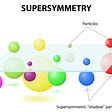## LOG or LN?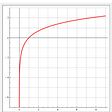## Quantum Logic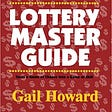## MINIMUM SPANNING TREE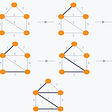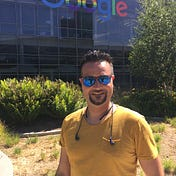## Batur Orkun

DevOps & Software & Architect & Linux Geek — http://baturorkun.com

## Recognizing Handwritten Digits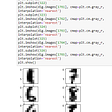## Supercomputers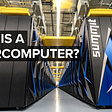## Data Structures And Algorithms in Machine Learning